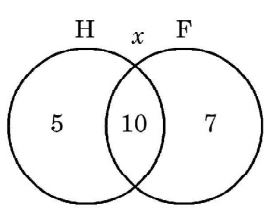## Numerical Ability

#### Numerical Ability

1. If 137+ 276 = 435 how much is 731 + 672?

1. By observation of summation, it can be seen that numbers are not in decimal. Let they have base n. Then converting all number in decimal
(137)n = [ 1 × n2 + 3n + 7n0 ]10
(276)n = [ 2n2 + 7n + 6n0 ]10
(435)n = [ 4n2 + 3n + 5n0 ]10
(731)n = [ 7n2 + 3n + 1n0 ]10
(672)n = [ 6n2 + 7n + 2n0 ]10
∴ (137)n + (276)n = (435)n
⇒ n2 + 3n + 7 + 2n2 + 7n + 6n = 4n2 + 3n + 5
⇒ n2 – 7n – 8 = 0 ⇒ n = – 1, 8
Possible base n = 8 (+ ve)
(435)n = (435)8 = [ 4 × 82 + 3 × 8 + 5 ]10 = (285)10
(731)n = (731)8 = [ 7 × 82 + 3 × 8 + 1 ]10 = (473)10
(672)n = (672)8 = [ 6 × 82 + 7 × 8 + 2 ]10 = (442)10
So , (731)8 + (672)8 = (473)10 + (442)10 = (915)10
(915)10 = (1623)8

##### Correct Option: C

By observation of summation, it can be seen that numbers are not in decimal. Let they have base n. Then converting all number in decimal
(137)n = [ 1 × n2 + 3n + 7n0 ]10
(276)n = [ 2n2 + 7n + 6n0 ]10
(435)n = [ 4n2 + 3n + 5n0 ]10
(731)n = [ 7n2 + 3n + 1n0 ]10
(672)n = [ 6n2 + 7n + 2n0 ]10
∴ (137)n + (276)n = (435)n
⇒ n2 + 3n + 7 + 2n2 + 7n + 6n = 4n2 + 3n + 5
⇒ n2 – 7n – 8 = 0 ⇒ n = – 1, 8
Possible base n = 8 (+ ve)
(435)n = (435)8 = [ 4 × 82 + 3 × 8 + 5 ]10 = (285)10
(731)n = (731)8 = [ 7 × 82 + 3 × 8 + 1 ]10 = (473)10
(672)n = (672)8 = [ 6 × 82 + 7 × 8 + 2 ]10 = (442)10
So , (731)8 + (672)8 = (473)10 + (442)10 = (915)10
(915)10 = (1623)8

1. 25 persons are in a room. 15 of them play hockey, 17 of them play football and 10 of them play both hockey and football. Then the number of persons playing neither hockey nor football is

1.∴ 5 + 10 + 7 + x = 25 ⇒ x = 3

##### Correct Option: D∴ 5 + 10 + 7 + x = 25 ⇒ x = 3